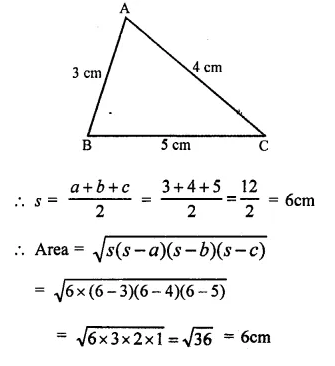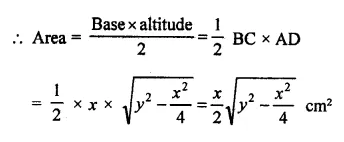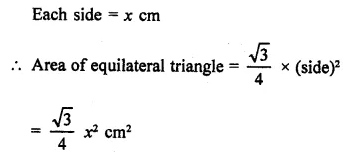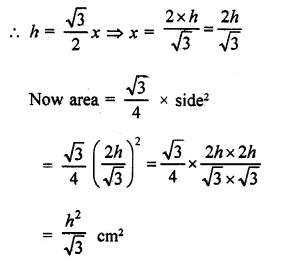## RD Sharma Class 9 Solutions Chapter 17 Constructions VSAQS

These Solutions are part of RD Sharma Class 9 Solutions. Here we have given RD Sharma Class 9 Solutions Chapter 17 Constructions VSAQS

Other Exercises

Question 1.
Find the area of a triangle whose base and altitude are 5 cm and 4 cm respectively.
Solution:
In ∆ABC,
Base BC = 5cmQuestion 2.
Find the area of a triangle whose sides are 3 cm, 4 cm and 5 cm respectively.
Solution:
Sides of triangle are 3 cm, 4cm and 5cmQuestion 3.
Find the area of an isosceles triangle having the base x cm and one side y cm.
Solution:
In isosceles ∆ABC,
AB = AC = y cm
BC = x cmQuestion 4.
Find the area of an equilateral triangle having each side 4 cm.
Solution:
Each side of equilateral triangle (a) = 4cmQuestion 5.
Find the area of an equilateral triangle having each side x cm.
Solution:Question 6.
The perimeter of a triangular field is 144 m and the ratio of the sides is 3 : 4 : 5. Find the area of the field.
Solution:
Perimeter of the field = 144 m
Ratio in the sides = 3:4:5
Sum of ratios = 3 + 4 + 5 = 12Question 7.
Find the area of an equilateral triangle having altitude h cm.
Solution:
Altitude of an equilateral triangle = h
Let side of equilateral triangle = xQuestion 8.
Let ∆ be the area of a triangle. Find the area of a triangle whose each side is twice the side of the given triangle.
Solution:
Let a, b, c be the sides of the original triangleHence area of new triangle = 4 x area of original triangle.

Question 9.
If each side of a triangle is doubled, then find percentage increase in its area.
Solution:
Sides of original triangle be a, b, cQuestion 10.
If each side of an equilateral triangle is tripled then what is the percentage increase in the area of the triangle?
Solution:
Let the sides of the original triangle be a, b, c and area ∆, thenHope given RD Sharma Class 9 Solutions Chapter 17 Constructions VSAQS are helpful to complete your math homework.

If you have any doubts, please comment below. Learn Insta try to provide online math tutoring for you.